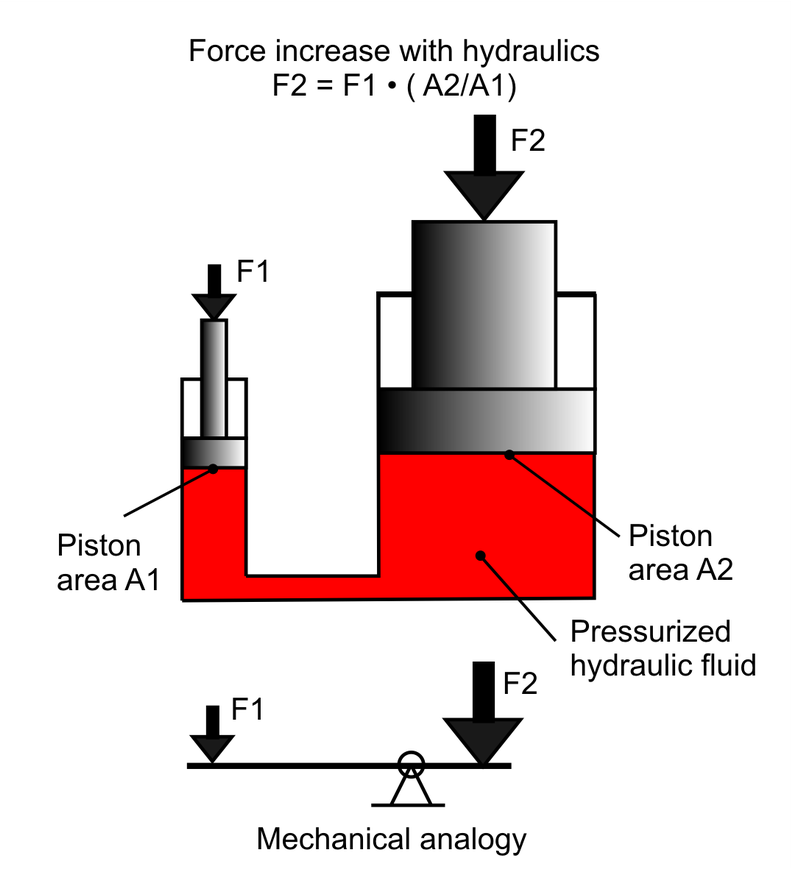# Hydraulic Pressure Calculator

By Dominik Czernia, PhD candidate
Last updated: May 28, 2020

This hydraulic pressure calculator analyzes a hydraulic system of two pistons connected to each other via a medium, usually a liquid. Such devices are usually used in situations where you have to lift something heavy using a lower force, e.g., automobile hydraulic lift in service stations. You can find mechanical analogy of hydraulic press in our mechanical advantage calculator, where we have described six simple machines such as a lever, a pulley or a wedge.

The basic principle of the hydraulic press is based on the so-called Pascal's law (also Pascal's principle). Read on if you want to learn what is Pascal's principle and how to use it to estimate appropriate areas of the pistons and the forces exerted on them. In the following text, we have also provided an example of hydraulics calculations which can be done with our Pascal's law calculator.

## What is Pascal's principle?

The Pascal's law says that if you apply external pressure on the liquid of gas in the enclosed container, the pressure will be transmitted throughout the fluid so that the same shift occurs everywhere. This law was named after Blaise Pascal who in 1646 performed an experiment called Pascal's barrel. He inserted a long vertical tube into a barrel filled with water. Then he poured the water into this tube, which increased the hydrostatic pressure. At some point, the pressure inside the barrel was too high and the barrel burst.

Pascal's principle is used in many hydraulic systems, such as hydraulic press, which is shown in the figure below. There are two pistons: one with a smaller area and one with a bigger area that are connected with each other via some hydraulic fluid. The pressure that the smaller piston exerts against the liquid will be precisely equal to the pressure the liquid exerts against the bigger cylinder. It is important to stress that not the forces, but pressures are equal.## Pascal's law calculator

Our hydraulic pressure calculator can compute suitable parameters of both pistons in the hydraulic press. To do this, we have used Pascal's law formula, which after appropriate transformations takes the form of:

`F₁ = A₁ / A₂ * F₂`

where

• `F₁` is the force applied to the first piston,
• `F₂` is the force applied to the second piston,
• `A₁` is the area of the first piston,
• `A₂` is the area of the second piston.

The pressure of the liquid inside the hydraulic press can be calculated in two simple ways: `p = F₁/A₁` or `p = F₂/A₂`. Although this is not obvious at first, the principle of energy conservation is fulfilled in the hydraulic press. To lift a heavier object lying on the first piston, we need to move the second piston at the longer distance. We can express it with these equations:

`d₁ = F₂ / F₁ * d₂` or `d₁ = A₂ / A₁ * d₂`

• `d₁` is the distance at which the first piston has moved,
• `d₂` is the distance at which the second piston has moved.

The total work done by one piston satisfies the relation:

`W = F₁ * d₁ = F₂ * d₂`

In the advanced mode of our hydraulic pressure calculator, you can also estimate parameters: `d₁`, `d₂` and `W`.

## Hydraulic calculations

Let's use our hydraulic press calculator to analyze automobile hydraulic lift. How much force do we need to apply to lift a car with the mass `1000 kg`? Let's go step by step:

1. Pressure is usually exerted on fluid in the small piston by a compressor. If you assume that the small piston is a circle with a diameter of `3 cm` then you easily compute that its area equals `A₁ = 7.069 cm²` (you can use our circle calculator to compute the area of the circle).
2. Similarly, if the bigger piston, which lifts the car, is a circle with `30 cm` in diameter then its area equals `A₂ = 706.9 cm²`.
3. The weight of car with mass of `1000 kg` is `F₂ = 1000 kg * 9.80665 m / s² = 98066.5 N`, because Earth's acceleration is `g = 9.80665 m / s²`.
4. Finally, with our Pascal's law calculator we can calculate that to lift a car with a mass of `1000 kg` we need only `F₁ = 980.7 N` which corresponds to the mass `100 kg`, approximately a mass of human body! At the same time, the liquid pressure is `p = 1387.3 kPa` which is `13.69` times higher than the atmospheric pressure. Check out pressure conversion calculator to learn how you can switch between different pressure units.
5. In the advanced mode of the hydraulic pressure calculator, you can also estimate how far a smaller piston should be pushed to lift the car, for example, at `10 cm`. After simple calculations, the answer is `10 m`, which is relatively long.
Dominik Czernia, PhD candidate
First piston
Area
in²
Force
N
Second piston
Area
in²
Force
N
Other
Liquid pressure
psi
People also viewed…

### Density altitude

The density altitude calculator tells you your effective altitude in terms of the International Standard Atmosphere, which is necessary for determining airplane engine performance and lift.

### Free fall

Our free fall calculator can find the velocity of a falling object and the height it drops from.

### Newton's law of cooling

The Newton's law of cooling calculator lets you find the time it takes for different objects to cool down.

### Titration

Use our titration calculator to determine the molarity of your solution.